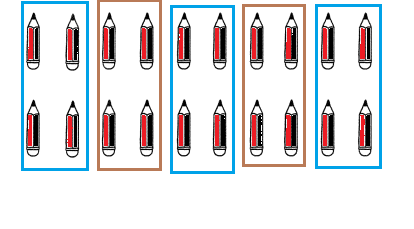# Math in Focus Grade 1 Chapter 18 Practice 2 Answer Key Sharing Equally

Go through the Math in Focus Grade 1 Workbook Answer Key Chapter 18 Practice 2 Sharing Equally to finish your assignments.

## Math in Focus Grade 1 Chapter 18 Practice 2 Answer Key Sharing Equally

Look at the pack of fish crackers. Then fill in the blanks.

Question 1.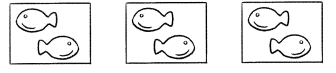There are ___________ fish crackers in all.
How many fish crackers are in each pack? ___________
There are 6 fish crackers in all.
2 fish crackers are in each pack.
Explanation:
Count the number of groups.
Then count the number of fishes in each group.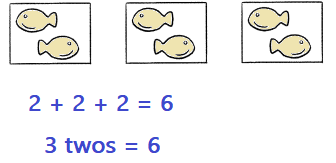Question 2.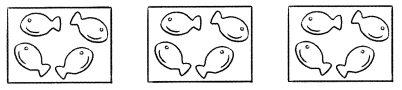There are ___________ fish crackers in all.
How many fish crackers are in each pack? ___________
There are 12 fish crackers in all.
Each pack there are 4 fishes,
Explanation:
Count the number of groups.
Then count the number of fishes in each group.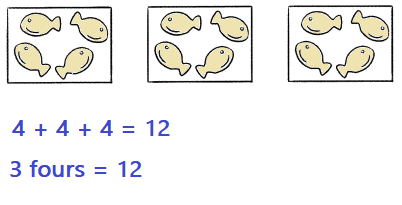Look at the pictures. Then fill in the blanks.

Question 3.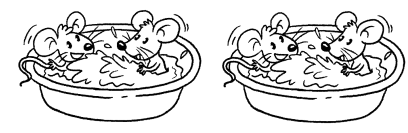There are ___________ mice in all.
There are ___________ tubs.
There are ___________ mice in each tub.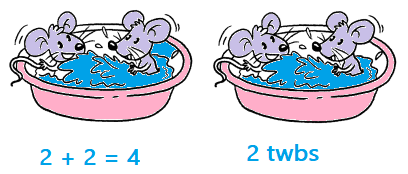There are 4 mice in all.
There are 2 tubs.
There are 2 mice in each tub.
Explanation:
Count the number of tubs = 2
Then count the number of mice in each tub.
2 + 2 = 4 mice.

Question 4.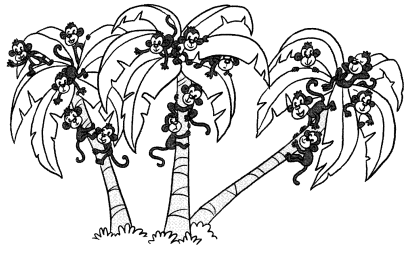There are ___________ monkeys in all.
There are ___________ trees.
There are ___________ monkeys on each tree.
15 monkeys.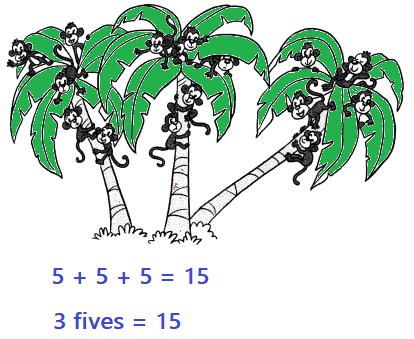There are 15 monkeys in all.
There are 3 trees.
There are 5 monkeys on each tree.
Explanation:
Count the number of trees = 3
Then count the number of monkeys on each tree.
5+5+5 = 15 monkeys.

Look at the pictures. Then fill in the blanks.

Question 5.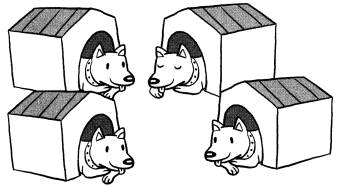There are ___________ dogs in all.
There are ___________ dog houses.
There is ___________ dog in each dog house.
There are 4 dogs in all.
There are 4 dog houses.
There is 1 dog in each dog house.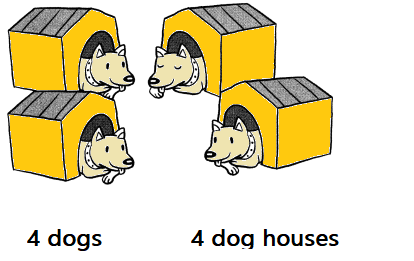Explanation:
Count the number of dog houses = 4
Then count the number of dogs in each dog house.
1+1+1+1 = 4 dogs

Question 6.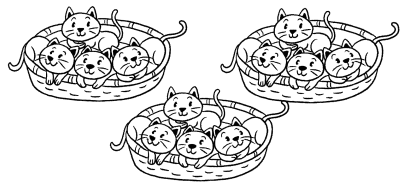There are ___________ cats in all.
There are ___________ cats in each basket.
There are 12 cats in all.
There are 4 cats in each basket.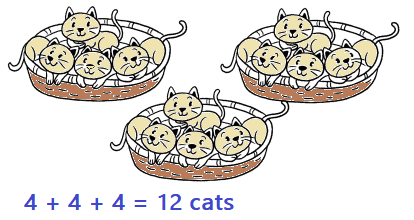Explanation:
Count the number of baskets are 4
Then count the number of cats in each basket.
4+4+4+4 = 12 cats

Fill in the blanks.

Dylan is cleaning the shoe rack. He has to put 8 shoes equally into 4 groups.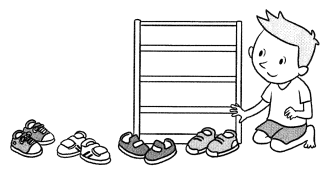He puts 1 shoe in each group.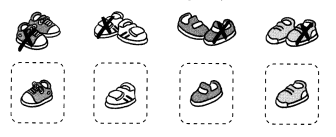Then he puts 1 more in each group.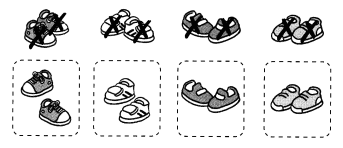Example
Each group has 2 shoes.

Fill in the blanks.

Question 7.
Martin is in the garden. He has to put 10 flowers equally into 2 groups.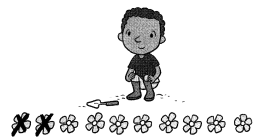Martin puts 2 books in each group. Help Martin put the rest of the books equally in each group.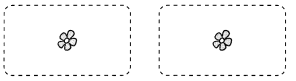Each group has ___________ flowers.
Each group has 5 flowers.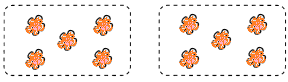Explanation:Explanation:
Count the number of groups = 2
Then count the number of flowers in each group.
5 + 5 = 10

Question 8.
Martin is in the library now. He has to put 15 books equally into 5 groups.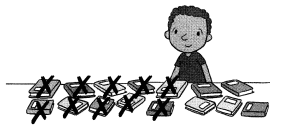Martin puts 2 books in each group. Help Martin put the rest of the books equally in each group.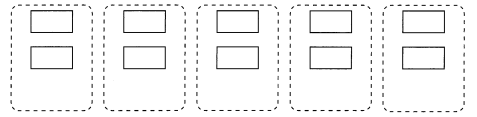Each group has ____________ books.
3 books
Explanation:
Count the number of groups.
Then count the number of books in each group.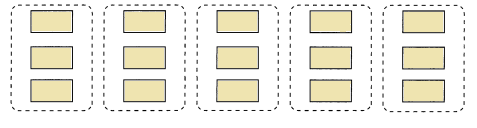5 x 3 = 15
3 + 3 + 3 + 3 + 3 = 15 books

Draw. Then fill in the blanks.

Question 9.
There are 12 peanuts. Draw an equal number of peanuts in each bag.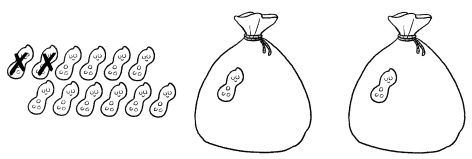There are ____________ in each bag.
6 peanuts in each bag.
Explanation:
Count the number of bags.
Then distribute the peanuts equally in 2 bags.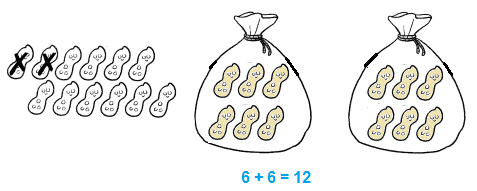Question 10.
There are 16 marbles. They are shared equally by 4 children. In each bag, draw an equal number of marbles for each child.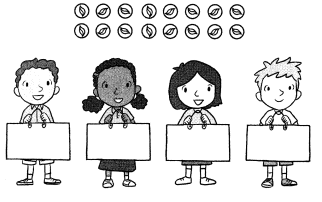Each child gets ____________ marbles.
Each child gets 4 marbles.
There are 16 marbles.
They are shared equally by 4 children.
So, each child gets 4 marbles.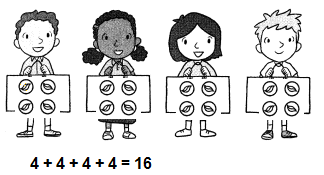Fill in the blanks.

Question 11.
There are 10 toys. Suki packs them into 5 boxes equally. How many toys are in each box?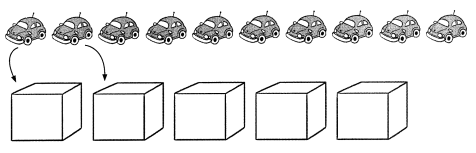There are ____________ toys in each box.
There are 2 toys in each box.
Explanation:
There are 10 toys.
Suki packs them into 5 boxes equally.
There are 2 toys in each box.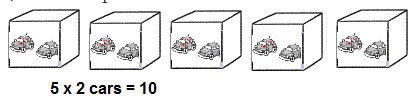Question 12.
There are 6 balloons. They are shared equally by 3 children. How many balloons does each child get?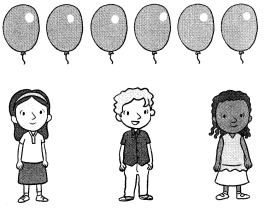Each child gets ____________ balloons.
Each child gets 2 balloons.
Explanation:
There are 6 balloons.
They are shared equally by 3 children.
Each child gets 2 balloons.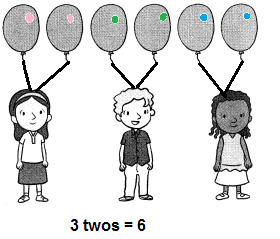Fill in the blanks.

Question 13.
Put 18 coins into 3 equal groups.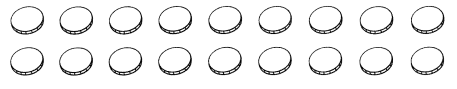There are ____________ coins in each group.
There are 6 coins in each group.
Explanation:
Count the number of coins = 18
Then divide these coins equally in 3 groups.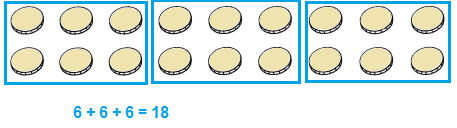Question 14.
Put 20 pencils info 5 equal groups.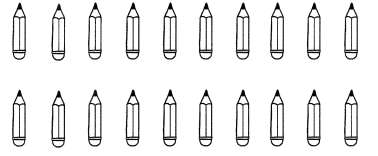There are ____________ pencils in each group.